# Decision Boundaries for Deep Learning and other Machine Learning classifiers

H2O, one of the leading deep learning framework in python, is now available in R. We will show how to get started with H2O, its working, plotting of decision boundaries and finally lessons learned during this series.

By Takashi J. OZAKI, Ph. D.

For a while (at least several months since many people began to implement it with Python and/or Theano, PyLearn2 or something like that), nearly I’ve given up practicing Deep Learning with R and I’ve felt I was left alone much further away from advanced technology…

But now we have a great masterpiece: {h2o}, an implementation of H2O framework in R. I believe {h2o} is the easiest way of applying Deep Learning technique to our own datasets because we don’t have to even write any code scripts but only to specify some of its parameters. That is, using {h2o} we are free from complicated codes; we can only focus on its underlying essences and theories.

With using {h2o} on R, in principle we can implement “Deep Belief Net”, that is the original version of Deep Learning*1. I know it’s already not the state-of-the-art style of Deep Learning, but it must be helpful for understanding how Deep Learning works on actual datasets. Please remember a previous post of this blog that argues about how decision boundaries tell us how each classifier works in terms of overfitting or generalization, if you already read this blog. :)

It’s much simple how to tell which overfits or well gets generalized with the given dataset generated by 4 sets of fixed 2D normal distribution. My points are: 1) if decision boundaries look well smoothed, they’re well generalized, 2) if they look too complicated, they’re overfitting, because underlying true distributions can be clearly divided into 4 quadrants with 2 perpendicular axes.

OK, let’s run the same trial with Deep Learning of {h2o} on R in order to see how DL works on the given dataset.

Datasets

Please get 3 datasets from my repository on GitHub:
simple XOR pattern, complex XOR pattern, and a grid dataset.

Github Repo for the current post. Of course, feel free to clone it; but any pull request will be rejected because this repository is not for software development. :P)

Getting started with {h2o} on R

First of all, H2O itself requires Java Virtual Machine environment. Prior to installing {h2o}, you have to install the latest version of Java SE SDK*2.

Next, {h2o} is not distributed via CRAN but available on GitHub. In order to install it, you have to add some arguments to run install.packages function.

```> install.packages("h2o",
repos=(c("http://s3.amazonaws.com/h2o-release/h2o/master/1542/R",
getOption("repos"))))
> library("h2o", lib.loc="C:/Program Files/R/R-3.0.2/library")
----------------------------------------------------------------------
Your next step is to start H2O and get a connection object (named
'localH2O', for example):
> localH2O = h2o.init()
For H2O package documentation, ask for help:
> ??h2o

After starting H2O, you can use the Web UI at http://localhost:54321

----------------------------------------------------------------------
At any rate, now you can run {h2o} in R.```

How {h2o} works on R

Once {h2o} package loaded, first you have to boot an H2O instance on Java VM. In the case below “nthreads” argument was set to -1, that means all CPU cores must be used for the H2O instance. If you want spare any cores, specify the number of cores you want to use for H2O, e.g. 7 or 6.

```> localH2O <- h2o.init(ip = "localhost", port = 54321, startH2O = TRUE,

H2O is not running yet, starting it now...

Note:  In case of errors look at the following log files:
C:\Users\XXX\AppData\Local\Temp\RtmpghjvGo/h2o_XXX_win_started_from_r.out
C:\Users\XXX\AppData\Local\Temp\RtmpghjvGo/h2o_XXX_win_started_from_r.err

java version "1.7.0_67"
Java(TM) SE Runtime Environment (build 1.7.0_67-b01)
Java HotSpot(TM) 64-Bit Server VM (build 24.65-b04, mixed mode)

Successfully connected to http://localhost:54321

R is connected to H2O cluster:
H2O cluster uptime:         1 seconds 506 milliseconds
H2O cluster version:        2.7.0.1542
H2O cluster name:           H2O_started_from_R
H2O cluster total nodes:    1
H2O cluster total memory:   7.10 GB
H2O cluster total cores:    8
H2O cluster allowed cores:  8
H2O cluster healthy:        TRUE```

Now you can run all functions of {h2o} package. Then load the simple XOR pattern and the grid dataset.

```> cfData <- h2o.importFile(localH2O, path = "xor_simple.txt")
> pgData<-h2o.importFile(localH2O,path="pgrid.txt")```

We’re ready to draw various decision boundaries using {h2o} package, in particular with Deep Learning. Let’s go to the next step.

Prior to trying Deep Learning, see the previous result

To compare a result of Deep Learning with ones of the other classifiers, please see the previous result. In the ones below, I ran decision tree, SVM with some sets of parameters, neural network (with only a hidden layer), and random forest.

#### Linearly inseparable and simple XOR pattern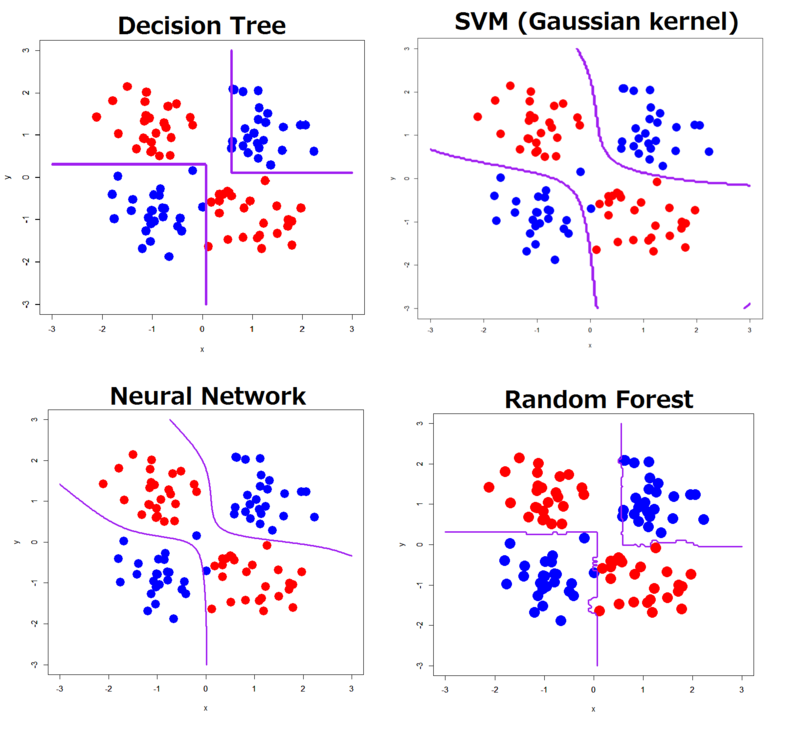As clearly seen, all of the classifiers showed decision boundaries well reflecting its true distribution.

#### Linearly inseparable and complex XOR pattern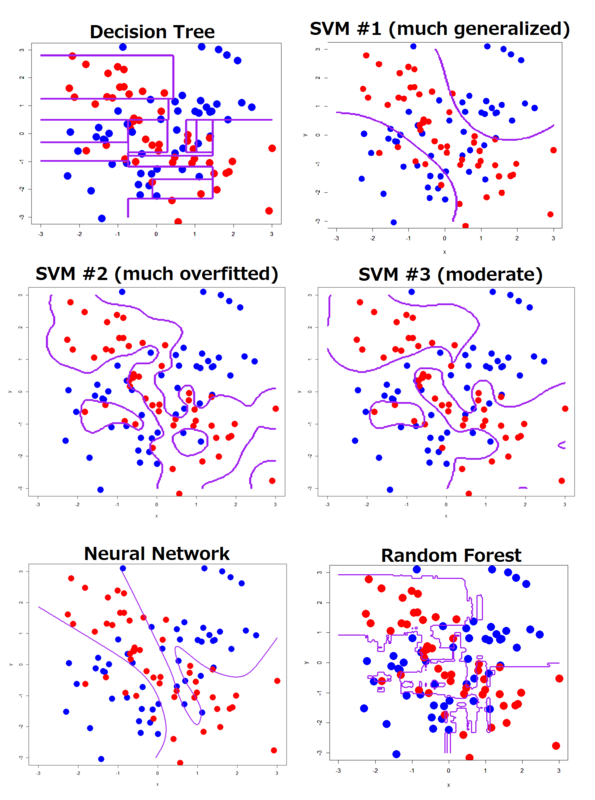In contrast to the simple XOR pattern, the result showed a wide variety of decision boundaries. Decision tree, neural network and random forest estimated much more complicated boundaries than the true boundaries, although SVM with well generalized by specific parameters gave natural and well smoothed boundaries (but classification accuracy was not good).

Drawing decision boundaries with {h2o}

OK, let’s run h2o.deeplearning function to estimate decision boundaries using Deep Learning.

Our primary interest here is what kind of set of tuning parameters shows what kind of decision boundaries. In h2o.deeplearning function, we can tune parameters (arguments) below:

• activation: Tanh, Rectifier and Maxout. We can also add “WithDropout” to implement the dropout procedure.
• hidden: details of hidden layers, by vector. c(3,2) means 1st hidden layer with 3 units and 2nd one with 2 units. rep(3,3) means 3 consecutive hidden layers with 3 units.
• epochs: the number of iteration. Of course larger epochs, more trained output you can get.
• autoencoder: logical value that determines whether autoencoder is used or not. Here we ignore it because the sample size is too small.
• hidden_dropout_ratio: dropout ratio of hidden layers by vector. Even if you want to implement the dropout procedure, we recommend to specify only 0.5 according to Baldi (NIPS, 2013).

For simplification, in this post I only tune “activation” and “hidden”. In particular about “hidden”, the number of hidden layers are fixed to 2 or 3 and the number of units are fixed to 5 or 10. Anyway we can run it as below.

```> res.dl<-h2o.deeplearning(x=1:2,y=3,data=xorsData,classification=T,
activation="Tanh",hidden=c(10,10),epochs=10)
> prd.dl<-h2o.predict(res.dl,newdata=pgData)
> prd.dl.df<-as.data.frame(prd.dl)
> plot(xors[,-3], pch=19, col=c(rep('blue',50), rep('red',50)),
cex=3, xlim=c(-4,4), ylim=c(-4,4), main="Tanh, (10,10)")
> par(new=T)
> contour(px, py, array(prd.dl.df[,1], dim=c(length(px), length(py))),
xlim=c(-4,4), ylim=c(-4,4), col="purple", lwd=3,drawlabels=F)```

#### simple XOR pattern with 2 hidden layers

Tanh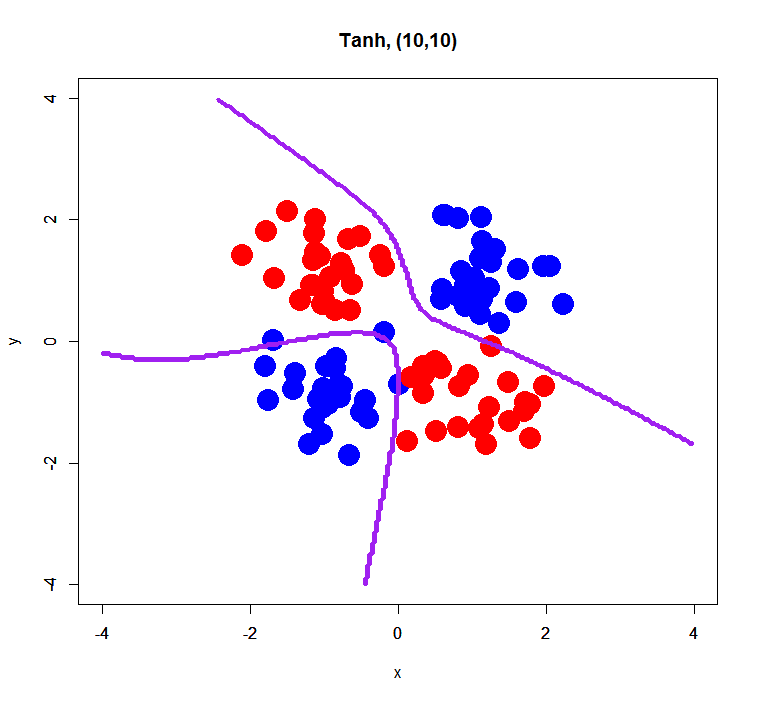Rectifier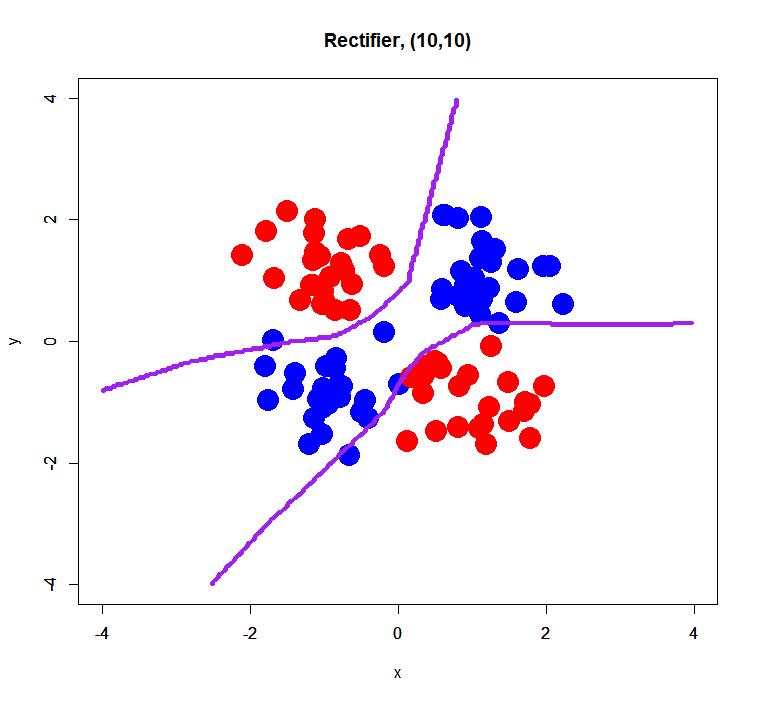Maxout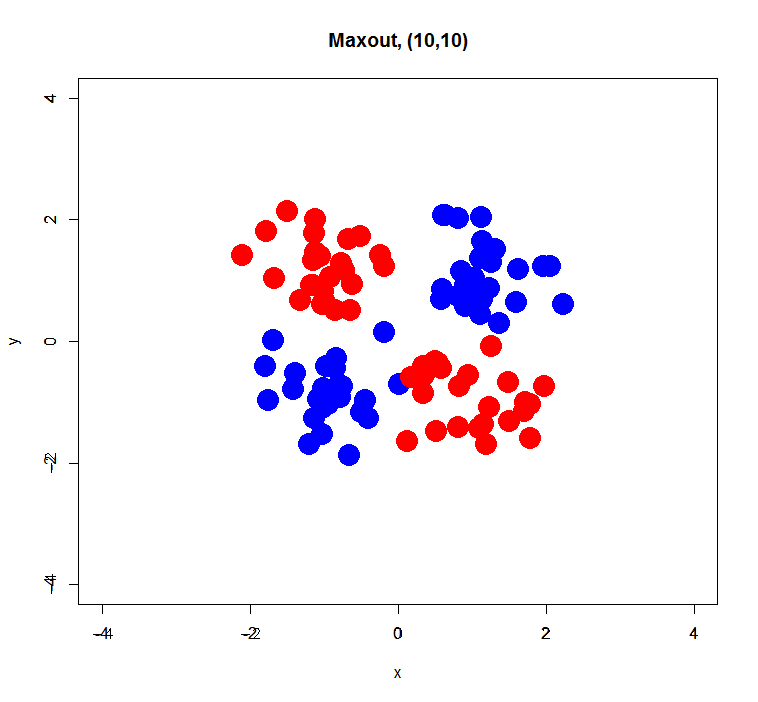Maxout failed to estimate a classification model correctly… perhaps it was caused by too small sample size (only 100) or too small dimension (just 2D). On the other hand, Tanh and Rectifier showed fairly good decision boundaries.

#### complex XOR pattern with 2 hidden layers

Tanh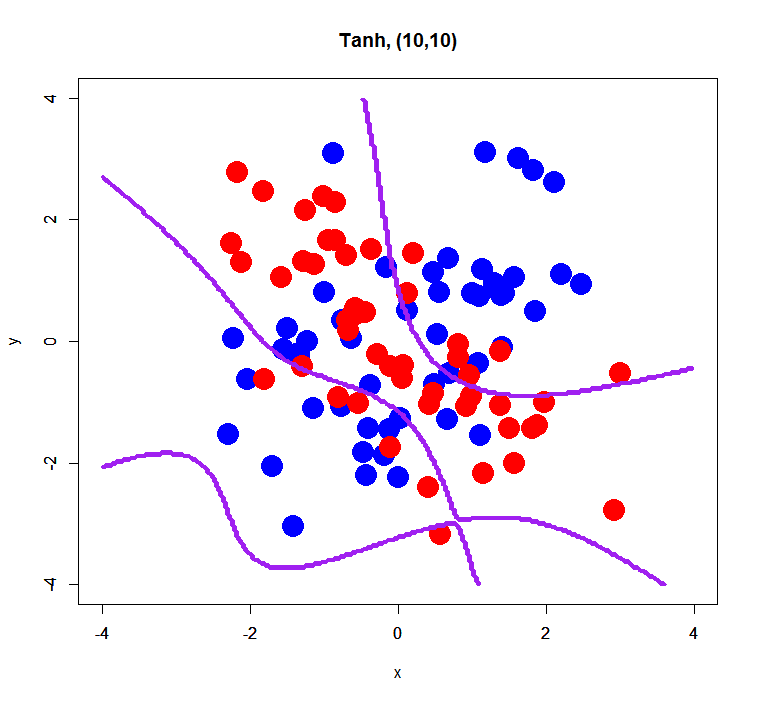Rectifier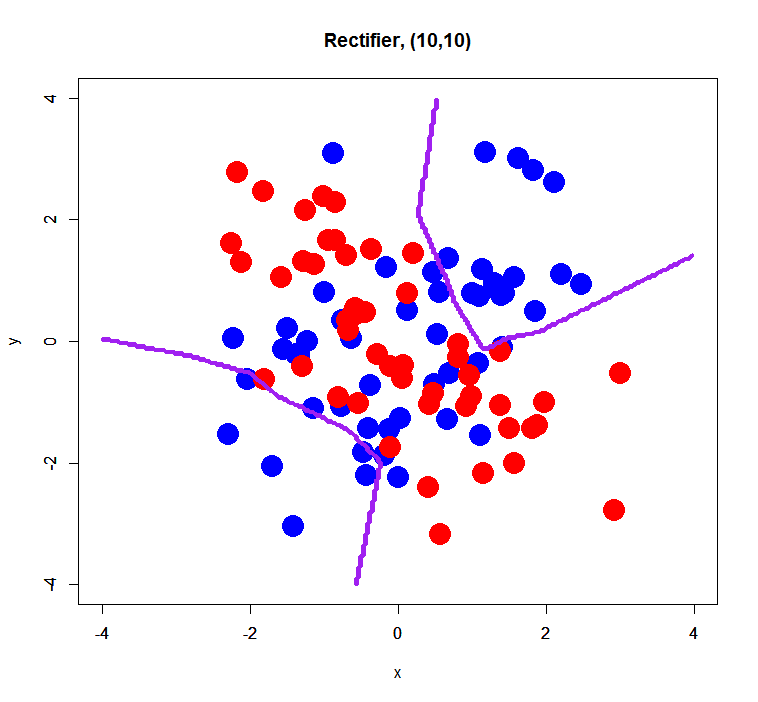In contrast to the result of the simple XOR pattern, Rectifier worked better than Tanh and its decision boundary is similar to the one of SVM (well generalized version). Tanh looks overfitting.

#### simple XOR pattern with 3 hidden layers

This is just a trial for evaluating an effect of the number of hidden layers. Prior to this trial, I think its number may affect a bit results of classification… so, how was it?

Tanh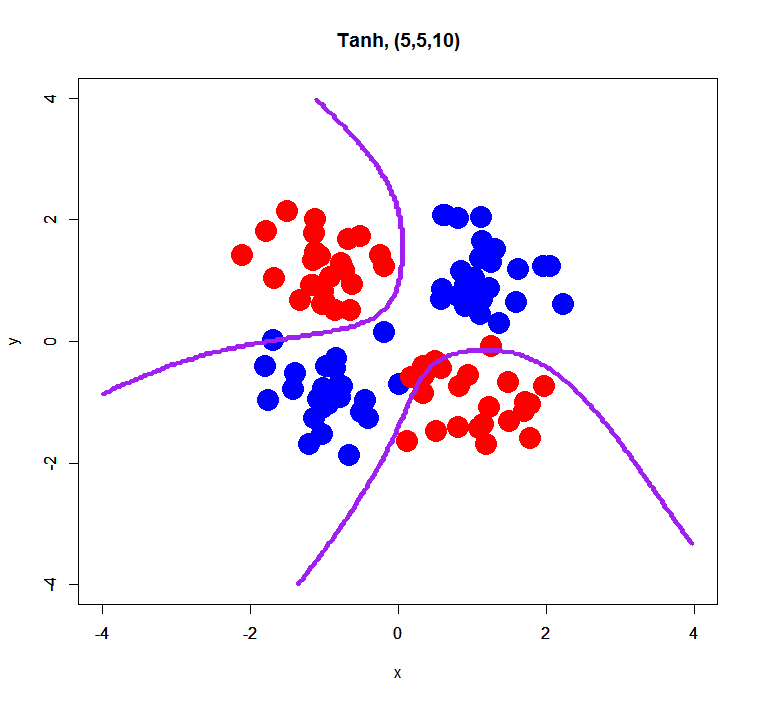Rectifier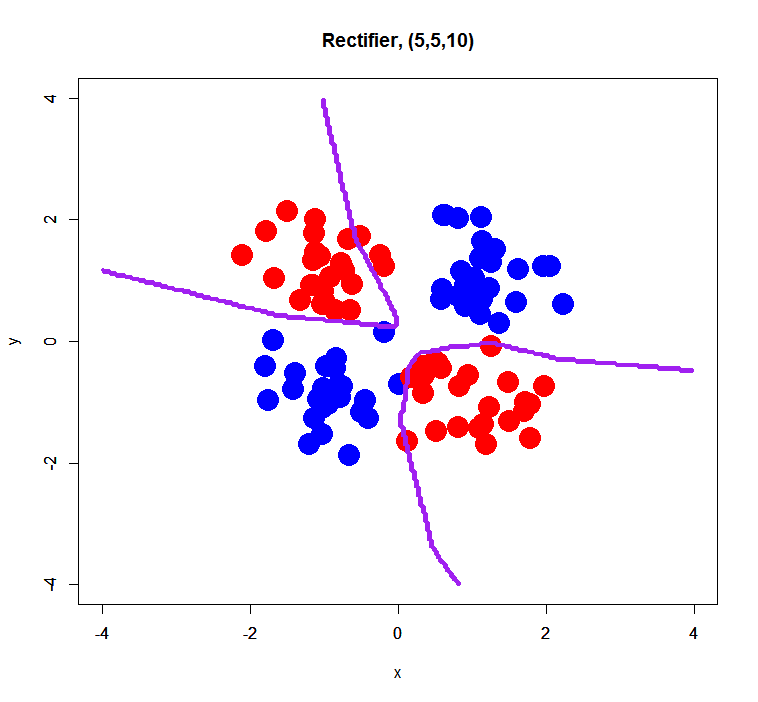Both of decision boundaries look getting more overfit than ones with 2 hidden layers, but seem to well classify samples.

#### complex XOR pattern with 3 hidden layers

Tanh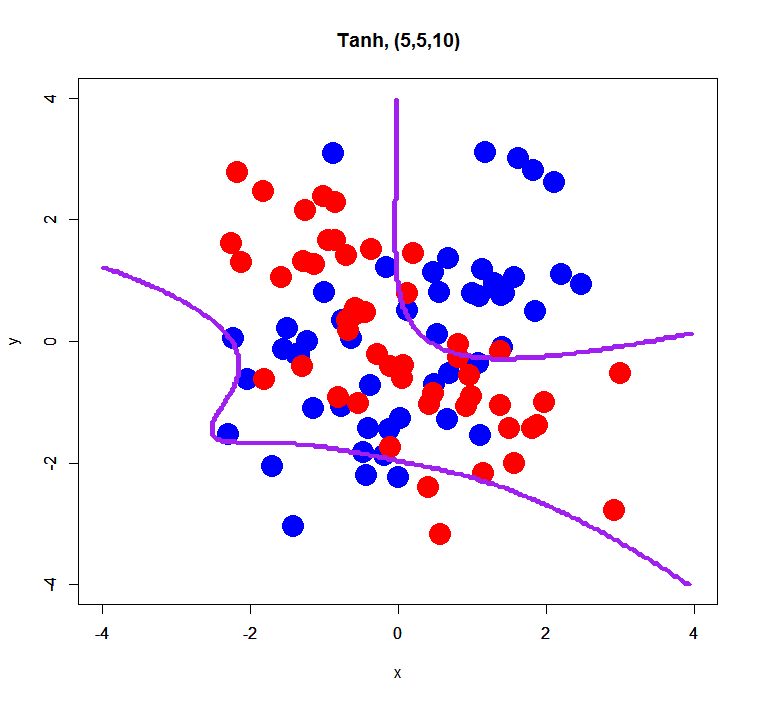Recitifier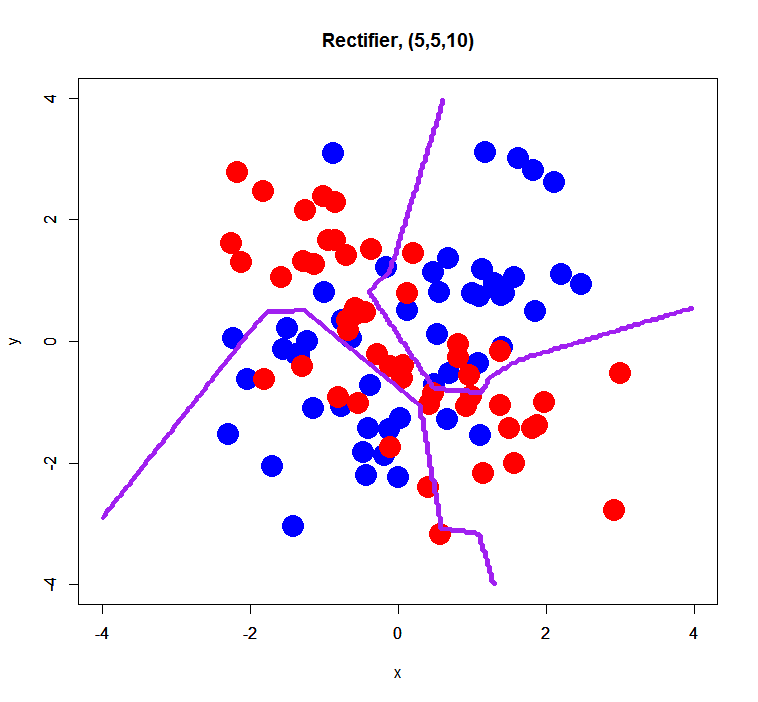I feel like joking. :) Tanh looks almost never working. Rectifier classified well but seems less generalized although its decision boundary looks not so overfitting.

Conclusion

The most important lesson that I learned from a series of these trials is that performance of Deep Learning would strongly depend on parameter tuning, including choosing which activation function, the number of hidden layers and/or the number of units of each layer.

I think this feature has been also known as an important feature of traditional neural network (with only a hidden layer). From the result here, I guess its characteristics are taken over by Deep Learning, although hidden layers in Deep Learning in early layers behave just as “preprocesser” or “feature generator” but not conventional classifiers.

My conclusion here is simple; be much careful about parameter tuning for Deep Learning. It can easily boost your result, but at the same time it can spoil your classifier.

• *1:Of course here I think the latest topic of Deep Learning is “ConvNet” or convolutional neural network with convolution and max pooling
• *2:I believe almost all of readers of this blog already installed it…

Bio: Takashi J. OZAKI, Ph. D. is a Data scientist, Quant analyst & researcher.

Original, reposted by permission.

Related: